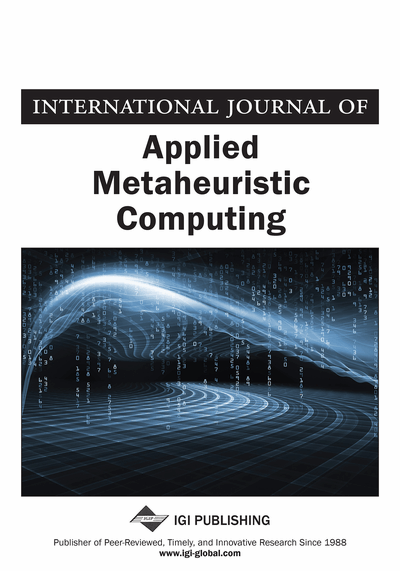# A Generalized Graph Strict Strong Coloring Algorithm

Mourad Bouzenada (MISC Laboratory, University Mentouri Constantine, Algeria), Meriem Bensouyad (MISC Laboratory, University Mentouri Constantine, Algeria), Nousseiba Guidoum (MISC Laboratory, University Mentouri Constantine, Algeria), Ahmed Reghioua (MISC Laboratory, University Mentouri Constantine, Algeria) and Djamel-eddine Saïdouni (MISC Laboratory, University Mentouri Constantine, Algeria)
DOI: 10.4018/jamc.2012010103
Available
\$37.50
No Current Special Offers

## Abstract

This paper examines the graph coloring problem. A graph strict strong coloring algorithm has been proposed for trees in Haddad and Kheddouci (2009). In this paper, the authors propose a new heuristic based algorithm for general graphs named GGSSCA (for Generalized Graph Strict Strong Coloring Algorithm). The authors show that the complexity of this algorithm is polynomial with considering the number of vertices.
Article Preview
Top

## 1. Introduction

The graph coloring is a combinatorial optimization problem. The coloring process is done according to some properties. Let G be a given graph. A proper vertex coloring of a graph is a vertex coloring such that no two adjacent vertices have the same color. The minimum number of colors among all proper colorings of G is its chromatic number χ(G). It is well known that the k-coloring problem for general graphs is NP-complete and the calculation of the chromatic number is NP-hard (Klotz, 2002).

A plenty variety of graph coloring is presented in Chen, Gyarfas, and Schelp (1998). Some others colorings deal with edges of graphs (Caragiannisa, Kaklamanisa, & Persianob, 2002), others with lists (Albertson, Grossman, & Haas, 2000). In addition too, solutions for coloring both vertices and edges of graphs have been proposed (Borodin, Kostochka, & Woodall, 1998).

Often, graph coloring and dominance problems are correlated. Several relations between the chromatic number and some dominance parameters are presented in Chellali and Volkmann (2004).

In Zverovich (2006), a notion of graph strong coloring has been introduced. It defines a dominance relation between graph vertices and color classes. Strong coloring problem has been shown as NP-complete.

After that, in Haddad and Kheddouci (2009), authors defined a strict strong k-coloring (k-SSColoring) of graphs, which is a strong k-coloring with a non-empty constraint on color classes. The new coloring parameters define the dominance property. An exact polynomial time of graph strict strong coloring algorithm for trees has been proposed. Graph Strict Strong Coloring Problem (GSSCP) consists to find the minimum number of colors which check the dominance property for each vertex. In fact, finding exact solutions for general graphs are NP-complete.

As an application of such coloring process we can cite broadcasting (Haddad, Dekar, & Kheddouci, 2008), data dissemination, gossiping.

In this paper, we show that heuristic based algorithms may be a good approach to solve graph strict strong coloring problem for general graphs.

The proposed algorithm is named GGSSCA (Generalized Graph Strict Strong Coloring Algorithm). The complexity of this algorithm is polynomial with respect to the number of vertices. The strict strong chromatic number χss(G) is the minimal number of colors needed to color properly its vertices such that each vertex of G is adjacent to at least one non empty color class (Haddad & Kheddouci, 2009) (Figure 1).

The paper is organized as follows: Section 2 gives some preliminary notations and definitions. In Section 3, the proposed algorithm is described. In Section 4, experimental results are presented. In Section 5, the proposed algorithm is applied on trees and results are compared with those of the exact algorithm. Conclusion and future work are given in the last section.

Top

## 2. Preliminary Defenitions

In this paper, we mean by graph a simple undirected loopless graph with no isolated vertices.

Let G = (V, E) be a graph such that V = {v1, v2... vn} is a finite set of vertices and E ⊆ V × V is a finite set of edges.

Two vertices u and v are neighbors or adjacent if (u, v) ∈ E.

For any u ∈ V, let N (u) = {v ∈ V such that (u, v) ∈ E} be the set of adjacent vertices of u. Let deg (u) = |N (u)| be the degree of the vertex u. A subset of vertex S ⊆ V is said stable or independent if and only if for any vertices u and v of S, (u, v) ∉ E. In other words, elements of S are not adjacent vertices.

## Complete Article List

Search this Journal:
Reset
Volume 13: 4 Issues (2022): 2 Released, 2 Forthcoming
Volume 12: 4 Issues (2021)
Volume 11: 4 Issues (2020)
Volume 10: 4 Issues (2019)
Volume 9: 4 Issues (2018)
Volume 8: 4 Issues (2017)
Volume 7: 4 Issues (2016)
Volume 6: 4 Issues (2015)
Volume 5: 4 Issues (2014)
Volume 4: 4 Issues (2013)
Volume 3: 4 Issues (2012)
Volume 2: 4 Issues (2011)
Volume 1: 4 Issues (2010)
View Complete Journal Contents Listing